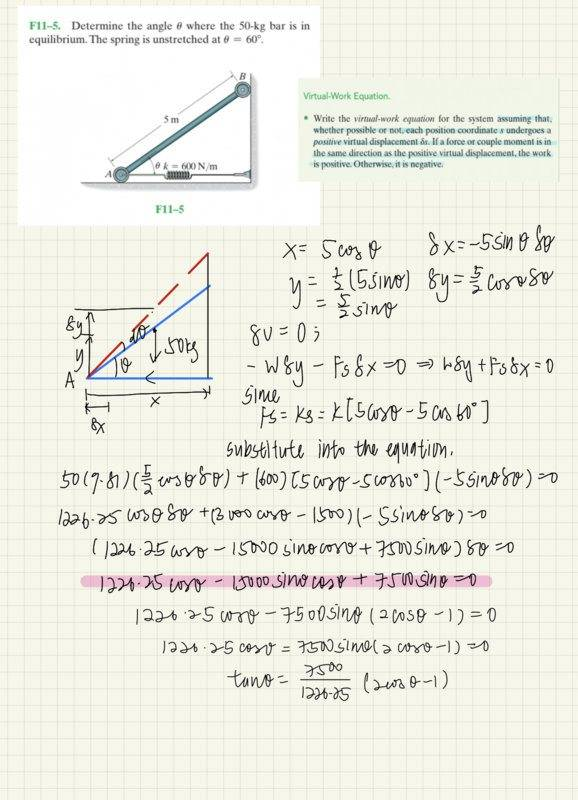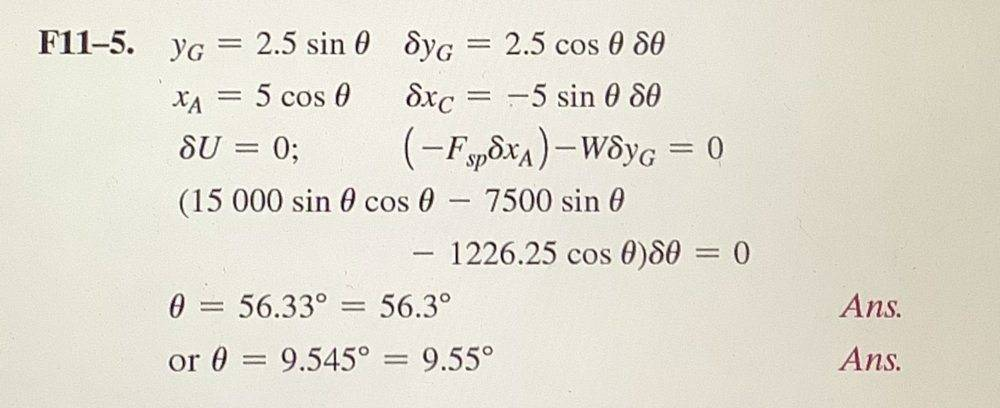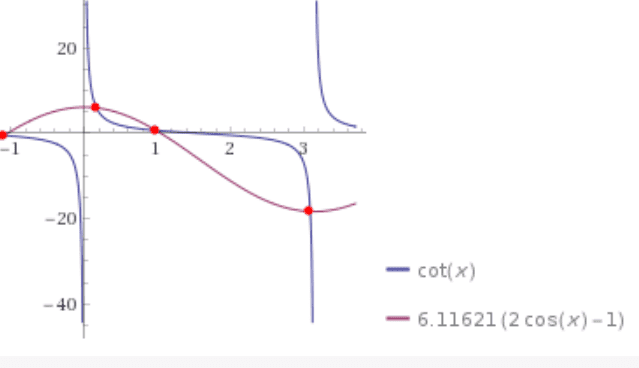# Solve the trigonometric equation involve sin(x), cos(x) and sin(x)cos(x)

daphnelee-mh
Homework Statement:
I had problem with solving the equation while doing my mechanics homework

How to solve equation : 1226.25cosx - 15000sinxcosx + 7500sinx = 0
Relevant Equations:
sin^2 + cos^2 = 1I can’t get the angle, answer given is x=56.33 , x=9.545. (All steps before the equation are correct.)

## Answers and Replies

Homework Helper
I can’t get the angle, answer given is x=56.33 , x=9.545.
You mean ##\theta = 0.983074## or ##\theta = 56.326^\circ##. Not sure what the x=9.545 means.
All steps before the equation are correct
Confirmed.
Aren't you in a situation where a numerical answer is adequate ? I don't think an analytical answer is possible.

Side note: LHS of your last equation should be a cotangent.

•etotheipi
I tried letting ##u = \cos{\theta}## and ended up with a quartic in ##u##, which my calculator could solve (I think it uses the quartic formula), but I certainly wouldn't have the patience to do that myself!

daphnelee-mh
You mean ##\theta = 0.983074## or ##\theta = 56.326^\circ##. Not sure what the x=9.545 means.
Confirmed.
Aren't you in a situation where a numerical answer is adequate ? I don't think an analytical answer is possible.

Side note: LHS of your last equation should be a cotangent.

daphnelee-mhGold Member
Hi daphne:

The following may be of some additional help.

sin 2x = 2 sin x cos x

Regards,
Buzz